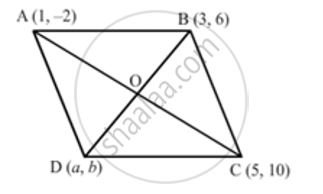# If Three Consecutive Vertices of a Parallelogram Are (1, -2), (3, 6) and (5, 10), Find Its Fourth Vertex. - Mathematics

If three consecutive vertices of a parallelogram are (1, -2), (3, 6) and (5, 10), find its fourth vertex.

If three consecutive vertices of a parallelogram ABCD are A (1,-2) , B (3,6) and C(5,10)  find its fourth vertex D.

#### Solution 1

Let ABCD be a parallelogram in which the coordinates of the vertices are A (1,−2);

B (3, 6) and C(5, 10). We have to find the coordinates of the fourth vertex.

Let the fourth vertex be D(x,y)

Since ABCD is a parallelogram, the diagonals bisect each other. Therefore the mid-point of the diagonals of the parallelogram will coincide.

Now to find the mid-point P(x,y) of two points A(x_1, y_1) and B(x_2,y_1) we use section formula as,

P(x,y) = ((x_1 + x_2)/2,(y_1+ y_2)/2)

The mid-point of the diagonals of the parallelogram will coincide.

So,

Co-oridinate of mid-point of AC = Co-ordinate of mid-point of BD

Therefore,

((5+1)/2, (10-2)/2) = ((x+3)/2, (y + 6)/2)

((x + 3)/2 ,(y + 6)/2) = (3,4)

Now equate the individual terms to get the unknown value. So,

(x + 3)/2= 3

Similarly

(y + 6)/2 = 4

y =  2

So the forth vertex is D(3,2)

#### Solution 2

LetA (1,-2) , B (3,6) and C(5,10)  be the three vertices of a parallelogram ABCD and the fourth vertex be D (a, b).

Join AC and BD intersecting at O.We know that the diagonals of a parallelogram bisect each other Therefore, O is the midpoint of AC as well as BD.

" Midpoint  of AC "=((1+5)/2 , (-2+10)/2) = (6/2,8/2) = (3,4)

"Midpoint  of BD "= ((3+a)/2 , (6+b)/2)

Therefore  , (3+a)/2 = 3 and (6+b)/2 = 4

⇒ 3+a =6 and 6+b=8

⇒ a = 6-3 and b = 8 -6

⇒ a= 3 and b = 2

Therefore, the fourth vertex is D (3,2) .

Concept: Coordinate Geometry
Is there an error in this question or solution?
Chapter 6: Co-Ordinate Geometry - Exercise 6.3 [Page 30]

#### APPEARS IN

RD Sharma Class 10 Maths
Chapter 6 Co-Ordinate Geometry
Exercise 6.3 | Q 49 | Page 30
RS Aggarwal Secondary School Class 10 Maths
Chapter 16 Coordinate Geomentry
Exercises 2 | Q 26

Share